Question

# Job А 3. The jobs listed below needed to be completed. Assume that it is now...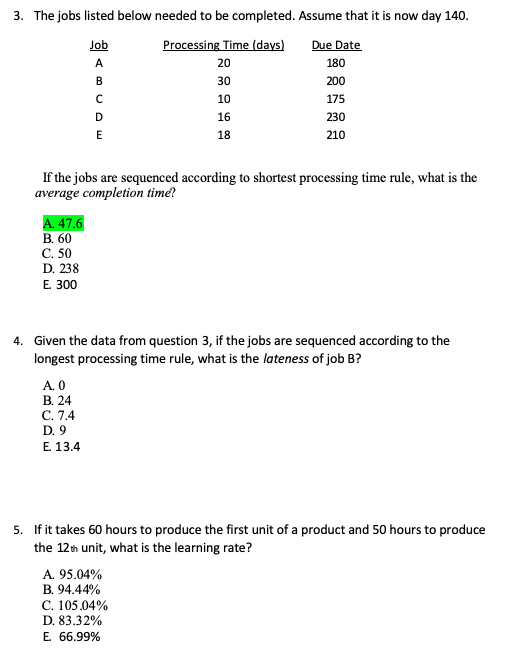Job А 3. The jobs listed below needed to be completed. Assume that it is now day 140. Processing Time (days) Due Date 20 180 30 200 10 175 230 210 B с D 16 18 E If the jobs are sequenced according to shortest processing time rule, what is the average completion time? A. 47.6 B. 60 C. 50 D. 238 E 300 4. Given the data from question 3, if the jobs are sequenced according to the longest processing time rule, what is the lateness of job B? A. O B. 24 C. 7.4 D. 9 E 13.4 5. If it takes 60 hours to produce the first unit of a product and 50 hours to produce the 12th unit, what is the learning rate? A. 95.04% B. 94.44% C. 105.04% D. 83.32% E 66.99%

3.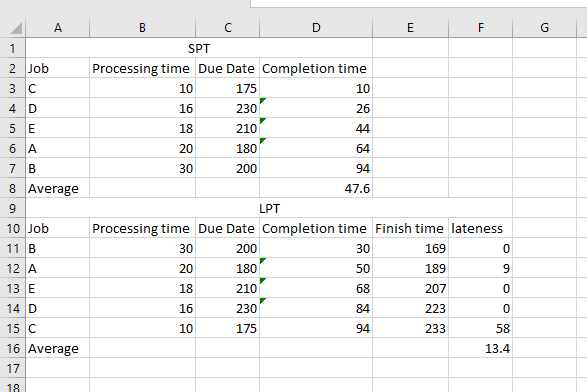Formula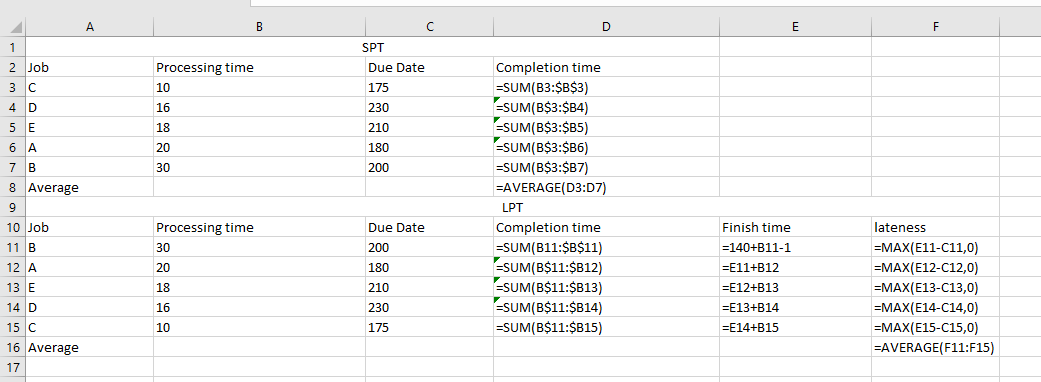Average completion time using SPT rule = 47.6 . Correct answer is A.

4.

Average lateness using LPT rule = 13.4. Correct answer is E.

5.

We know, Tn = T1*n^(ln(l)/ln(2)) where Tn = time required to complete nth unit and l = learning rate

For n = 12,

50 = 60*12^(ln(l)/ln(2))

or, 0.833333333 = 12^(ln(l)/ln(2))

Substituting value of options we get following set of values for RHS among which for learning rate = 95.04%, LHS = RHS approximately. option A is matching closely

So. correct answer is A. 95.04%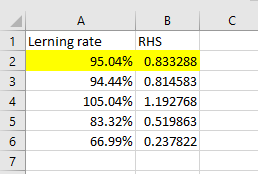Formula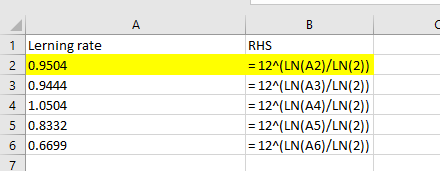#### Earn Coins

Coins can be redeemed for fabulous gifts.

Similar Homework Help Questions
• ### А 3. The jobs listed below needed to be completed. Assume that it is now day...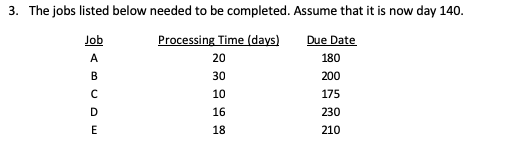А 3. The jobs listed below needed to be completed. Assume that it is now day 140. Job Processing Time (days) Due Date 20 180 30 200 10 175 16 230 18 210 B с D E 4. Given the data from question 3, if the jobs are sequenced according to the longest processing time rule, what is the lateness of job B? A. O B. 24 C. 7.4 D. 9 E 13.4 5. If it takes 60 hours to...

• ### # A 3. The jobs listed below needed to be completed. Assume that it is now...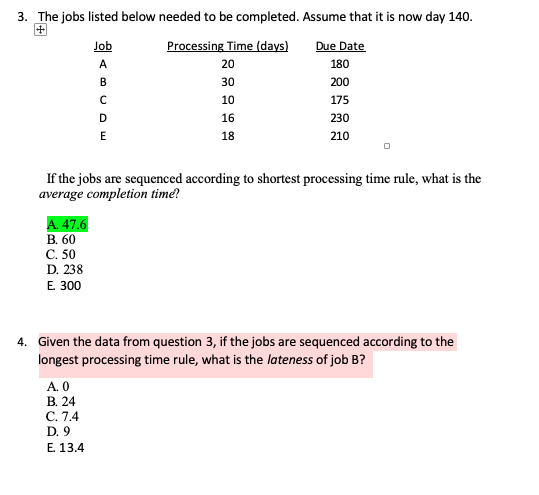# A 3. The jobs listed below needed to be completed. Assume that it is now day 140. Job Processing Time (days) Due Date 20 180 30 с 10 175 16 230 E 18 210 B 200 D 1:54 If the jobs are sequenced according to shortest processing time rule, what is the average completion time! A. 47.6 B. 60 C. 50 D. 238 E 300 4. Given the data from question 3, if the jobs are sequenced according to...

• ### 6. If it took 80 hours to produce the first unit of a product, and the...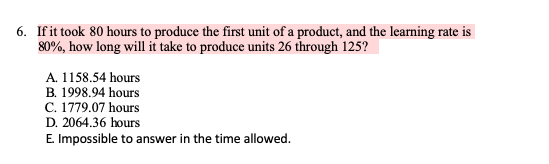6. If it took 80 hours to produce the first unit of a product, and the learning rate is 80%, how long will it take to produce units 26 through 125? A. 1158.54 hours B. 1998.94 hours C. 1779.07 hours D. 2064.36 hours E Impossible to answer in the time allowed. 5. If it takes 60 hours to produce the first unit of a product and 50 hours to produce the 12th unit, what is the learning rate? A. 95.04%...

• ### If the following jobs are sequenced according to the SLACK rule then the maximum job tardiness...

If the following jobs are sequenced according to the SLACK rule then the maximum job tardiness (in days) is (assume zero for today's date) Job Processing Time (days) Due Date A 8 12 B 6 15 C 11 17 D 7 10 E 3 8

• ### Processing times and due dates for five jobs are shown in the table below. Assume the...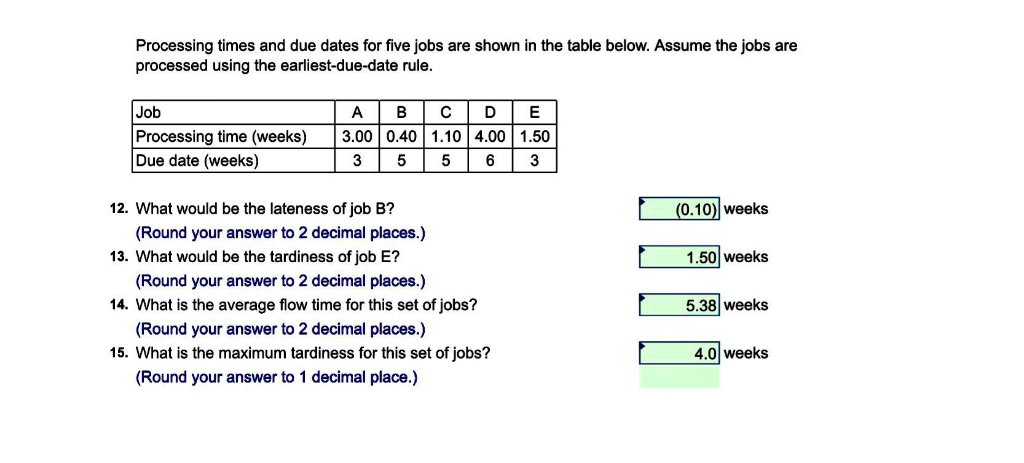Processing times and due dates for five jobs are shown in the table below. Assume the jobs are processed using the earliest-due-date rule Job Processing time (weeks) 3.00 0.40 1.10 4.00 1.50 Due date (weeks) 3 556 3 12. What would be the lateness of job B? 13. What would be the tardiness of job E? 14. What is the average flow time for this set of jobs? 15. What is the maximum tardiness for this set of jobs? (0.10...

• ### The following jobs are waiting to be processed at a small machine​ center: JOB DUE DATE...

The following jobs are waiting to be processed at a small machine​ center: JOB DUE DATE DURATION (DAYS) 10 240 18 20 232 14 30 240 6 40 245 30 50 255 26 All dates are specified as manufacturing planning calendar days. Assume that all jobs arrived on day 200 ​(yesterday) in the order shown but are not scheduled to begin until day 201 (today). a. Sequence the jobs according to LPT According to the LPT rule, the sequence of...

• ### a) Using the FCFS (first come, first served) decision e for sequencing the jobs, the order...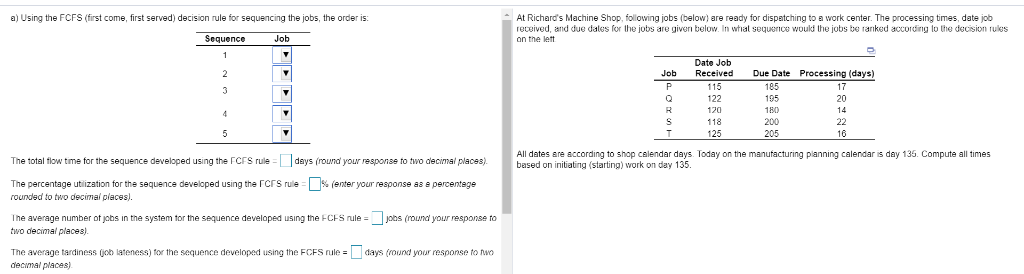a) Using the FCFS (first come, first served) decision e for sequencing the jobs, the order is t Richard's Machine Shop, following jobs (below) are ready for dispatching to a work center. The processing times, date job recuived, and due dales for the jobs a ranked according lo the decision rukes given below whal sequence would the jobs b Sequence Job Date Job Processing (days) Job Received D 105 122 195 20 180 120 205 25 I times d daysloday...

• ### The following jobs are waiting to be processed at a small machine​ center: JOB DUE DATE...

The following jobs are waiting to be processed at a small machine​ center: JOB DUE DATE DURATION (DAYS) 10 260 8 20 252 18 30 260 26 40 270 32 50 275 14 All dates are specified as manufacturing planning calendar days. Assume that all jobs arrived on day 200 ​(yesterday) in the order shown but are not scheduled to begin until day 201 (today). a. Sequence the jobs according to LPT? b. What is the average completion (flow) time?...

• ### a) Using the EDD (earliest due date) decision rule for sequencing the jobs, the order is...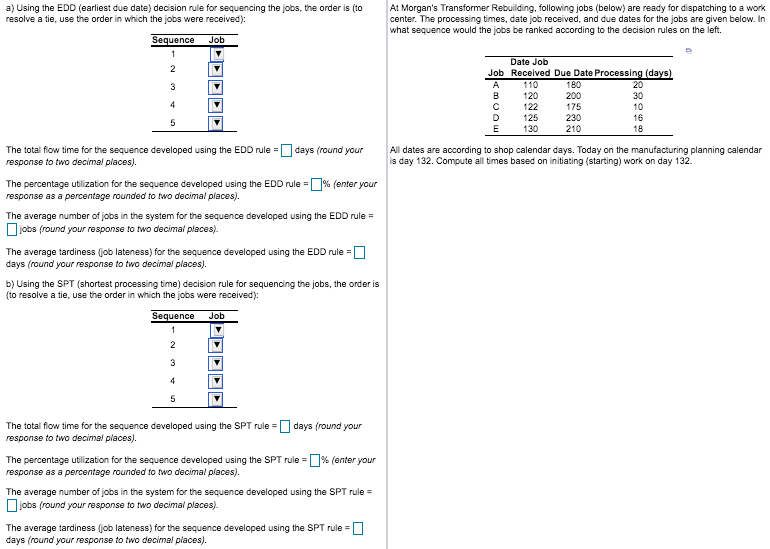a) Using the EDD (earliest due date) decision rule for sequencing the jobs, the order is (to resolve a tie, use the order in which the jobs were received): At Morgan's Transformer Rebuilding, following jobs (below) are ready for dispatching to a work center. The processing times, date job received, and due dates for the jobs are given below. In what sequence would the jobs be ranked according to the decision rules on the left Sequence Job 1 Date Job...

• ### a) Using the EDD (earliest due date) decision rule for sequencing the jobs, the order is...a) Using the EDD (earliest due date) decision rule for sequencing the jobs, the order is (to resolve a tie, use the order in which the jobs were received): At Morgan's Transformer Rebuilding, following jobs (below) are ready for dispatching to a work center. The processing times, date job received, and due dates for the jobs are given below. In what sequence would the jobs be ranked according to the decision rules on the left Sequence Job 1 Date Job...